## Problem statement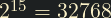and the sum of its digits is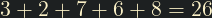.

What is the sum of the digits of the number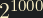?

## Thoughts

I tried for a while to find a pattern in the values, in the differences between each term, and even in different bases, but I haven't been able to find one (except for base 2 where the sum is always 1, or other powers of 2 which have regular oscillations). I think the way to go is just to compute the value.

## Solution

As with some previous problems, the number will be too big to fit in C's standard numeric types. It will be in the order of magnitude of at least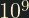, and will obviously need 1001 bits to store. I could import some library that handles big numbers, but I prefer to work it out myself.

To work out how many decimal digits we need, we can observe that it have order of magnitude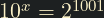, meaning we need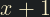digits.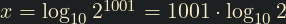.

To solve, we can use simple "long multiplication" as learned in primary school: from the smallest to largest digit, multiply by 2 and them sum the carry from the previous digit.

#include <stdio.h>
#include <math.h>

int main()
{
const int base = 2;
const int exponent = 1000;
const int num_digs = (exponent + 1) * log10(base) + 1;

char result[num_digs];

for (int i = 0; i < num_digs; i++)
result[i] = 0;

result = 1;

for (int i = 0; i < exponent; i++) {
int carry = 0;
int j = 0;
/* We can stop looping at j = i because multiplying any number by 2 can only
* increase the number of decimal digits by a maximum of 1 */
for (int j = 0; j <= i && j < num_digs; j++) {
int val = result[j] * base + carry;
result[j] = val % 10;
carry = val / 10;
}
}

int sum = 0;
for (int j = 0; j < num_digs; j++) {
sum += result[j];
}

printf("%d\n", sum);
}